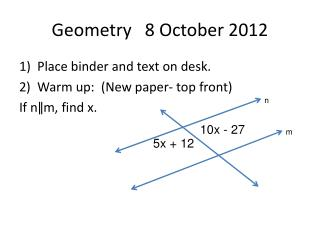DownloadDownload PresentationGeometry 8 October 2012

# Geometry 8 October 2012

Download Presentation## Geometry 8 October 2012

- - - - - - - - - - - - - - - - - - - - - - - - - - - E N D - - - - - - - - - - - - - - - - - - - - - - - - - - -
##### Presentation Transcript

1. Geometry 8 October 2012 • Place binder and text on desk. • Warm up: (New paper- top front) If nǁm, find x. n 10x - 27 m 5x + 12

2. Objective Students will use mathematical modeling to find the rule for the nth term. Students will take notes, do an investigation and work collaboratively. DUE TUESDAY: 2.3, pg. 112: 1 – 4, 6, 9, 10, 20 DISTRICT INTERIM TEST on Chapters 1 and 2 Wednesday and Thursday BRING COMPASS TO CLASS on Wed/ Th

3. Inductive Reasoning the process of OBSERVING FINDING PATTERNS MAKING GENERALIZATIONS… GEOMETRY the generalization is called A CONJECTURE!!! If……then………

4. Linear y = mx + b functions m = slope= b = y-intercept (y value when x = 0) FUNCTION f(x) = mx + b notationread as “f of x equals m x plus b” Function rule The rule that gives the nth term for a sequence is called the function rule.

5. linear equations Intercept form y = b + mx (OR y = mx + b ) of a linear function y = b ± mx y = b + mx Slope goes with the x 1. where does it start? y-intercept, value when x = 0 2. increasing or decreasing? 3. By how much? (add or subtract repeatedly x times)

6. CW: function notation IF f(n) = 3n – 2, find: 1) f(1) = 3(1) – 2 = 3 – 2 = 1 (substitute 1 for n) 2) f(2) 3) f(5) 4) f(10) 5) f(20) (or 20th term in a sequence) 6) f(100) (or 100th term in a sequence)

7. using your rule to predict Write your rule in function notation linear equation: y = 3n – 2 function rule: f(n) = 3n – 2 To predict the value of the 20th term, you can substitute 20 for n f(20) = 3(20) – 2 = 60 – 2 = 58 “the function evaluated when n is 20 equals….” “f at 20 equals…”

8. DID YOU FIND THE RULE?? Mathematical models used for the “# of handshake” problem: a table a set of points w/ connecting line segments sum of integers triangle of dots rule or formula WHICH MODEL is most useful for solving with any number of students? WHICH gives the most insight into the situation? WHICH leads to a formula using inductive reasoning?

9. rectangular numbers Read page 111. Strategy– if n is the number of the term in a sequence, use n for one dimension and try to express the 2nd dimension in terms of n.

10. Methods for solving quadratics from tables See handout “solving quadratics” 1) Try models- rectangle? points w/ segments? Tables? Sketches? Shared parts? 2) Table- find DIFFERENCES! 2nd difference constant? => quadratic! 3) Try n2 ± ??? 4) Look for patterns in the FACTORS of the values

11. debrief Which model for the handshake problem makes most sense to you? Why? How did it help you think about the problem?